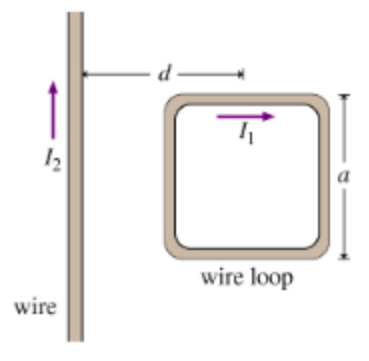# Problem: A square loop of wire with side length a carries a current I1. The center of the loop is located a distance d from an infinite wire carrying a current I2. The infinite wire and loop are in the same plane; two sides of the square loop are parallel to the wire and two are perpendicular as shown.Part A. What is the magnitude, F, of the net force on the loop? Express the force in terms of I1, I2, a, d, and μ0.Part B. The magnetic moment m→ of a current loop is defined as the vector whose magnitude equals the area of the loop times the magnitude of the current flowing in it (m = IA), and whose direction is perpendicular to the plane in which the current flows. Find the magnitude, F, of the force, F, of the force on the loop from Part A in terms of the magnitude of its magnetic moment. Express F in terms of m, I2, a, d, and μ0.

###### FREE Expert Solution

Part A

The force in the loop is obtained by summing up the forces produced by the two parallel wires in the loop.

The force on the left side of the loop is given by:

$\begin{array}{rcl}{\mathbf{F}}_{\mathbf{1}}& \mathbf{=}& \frac{{\mathbf{\mu }}_{\mathbf{0}}{\mathbf{i}}_{\mathbf{1}}{\mathbf{i}}_{\mathbf{2}}}{\mathbf{2}\mathbf{\pi }\mathbf{r}}\\ & \mathbf{=}& \frac{{\mathbf{\mu }}_{\mathbf{0}}{\mathbf{i}}_{\mathbf{1}}{\mathbf{i}}_{\mathbf{2}}\mathbf{a}}{\mathbf{2}\mathbf{\pi }\mathbf{\left(}\mathbf{d}\mathbf{-}\frac{\mathbf{a}}{\mathbf{2}}\mathbf{\right)}}\end{array}$

Force on the right side of the loop:

$\begin{array}{rcl}{\mathbf{F}}_{\mathbf{2}}& \mathbf{=}& \frac{{\mathbf{\mu }}_{\mathbf{0}}{\mathbf{i}}_{\mathbf{1}}{\mathbf{i}}_{\mathbf{2}}}{\mathbf{2}\mathbf{\pi r}}\\ & \mathbf{=}& \frac{{\mathbf{\mu }}_{\mathbf{0}}{\mathbf{i}}_{\mathbf{1}}{\mathbf{i}}_{\mathbf{2}}\mathbf{a}}{\mathbf{2}\mathbf{\pi }\mathbf{\left(}\mathbf{d}\mathbf{+}\frac{\mathbf{a}}{\mathbf{2}}\mathbf{\right)}}\end{array}$

79% (284 ratings)###### Problem Details

A square loop of wire with side length a carries a current I1. The center of the loop is located a distance d from an infinite wire carrying a current I2. The infinite wire and loop are in the same plane; two sides of the square loop are parallel to the wire and two are perpendicular as shown.Part A. What is the magnitude, F, of the net force on the loop? Express the force in terms of I1, I2, a, d, and μ0.

Part B. The magnetic moment $\stackrel{\to }{\mathrm{m}}$ of a current loop is defined as the vector whose magnitude equals the area of the loop times the magnitude of the current flowing in it (m = IA), and whose direction is perpendicular to the plane in which the current flows. Find the magnitude, F, of the force, F, of the force on the loop from Part A in terms of the magnitude of its magnetic moment. Express F in terms of m, I2, a, d, and μ0.